1. Short term volume indices: A) Laspeyres formula（LQ）Laspeyres short term volume index（LQ）equals value index（IV）divided by Paasche price index（Pp）, where p is price，q is quantity：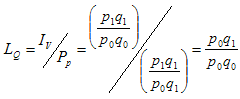Despite the changes in commodity set, Laspeyres index uses the price of the base year to calculate volume and its changes. B) Paasche formula（PQ）Paasche short term volume index（PQ）equals value index（IV）divided by Laspeyres price index（LP）。：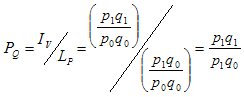Paasche volume index on the other hand uses the price of the current year to calculate volume and its changes. C) Fisher formula（FQ）Fisher index is the geometric mean of Laspeyres and Passche indices, formula as follows: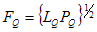Fisher volume index integrates the Laspeyres and Passche volume indices; it yields results that lie between the boundaries of the Laspeyres and Passche indices, and resolves the shortcomings of both indices, thus is called the ideal index. D) Reasons for choosing Laspeyres indexSubstantive mathematical proofs show that Laspeyres index overstates the true growth whereas Paasche index understates the true growth; meanwhile, Fisher index lies between Laspeyres and Paasche indices, and it theoretically provides the best measure of growth. Nevertheless, the above mathematical formulae show that complete information on prices and quantities in the current year are required to calculate either Paasche or Fisher indices. It takes time to collect complete information on prices and quantities in the current year, accurate forecast of prices and quantities in the current year are required to minimize the discrepancy in the latest economic growth. It becomes even more complicated and difficult for quarterly estimates as seasonal adjustment is needed. Therefore, even though Fisher index is theoretically superior, due to timeliness and accuracy of forecast, the United Sates and Canada are the only countries that use Fisher index to calculate chain volume measures. Paasche index is not being used due to timeliness as well as a less accurate result than Fisher index. When chain volume measures are used to calculate GDP, the discrepancies of either Laspeyres or Paasche indices are being reduced, i.e., the result is getting closer to Fisher index. Therefore, most countries (including the European Union, Australia, New Zealand, Hong Kong) that adopt chain volume measures, use Laspeyres index to calculate short term volume index in consideration of timeliness, accuracy and costs. 2. Chain volume index formula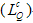Laspeyres volume index is used to compile chain volume index. Formula for chain volume index are different preceding and following the reference yearFormula following the reference year: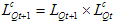Assume t is the reference year,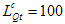Therefore,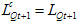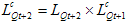Formula preceding the reference year: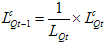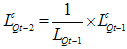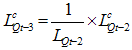The formulae for other years are derived in the same way.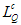is chain volume index,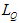is short term volume index, t is the reference year.Chain volume indices derived with the abovementioned formulae form a series of chain volume indices with reference year equal to 100. 3. Chain volume measures formula (Vn) This formula is used to compile chain volume measures. Users prefer to use value for economic analysis and comparison; therefore, chain volume indices series are chain-linked to the current price of the reference year to derive the chain volume measures in terms of the price of the reference year. Formula as follows: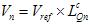where Vn is the chain volume measure in year n, Vref is the chain volume measure in the reference year (equal to current price),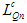is the chain volume index in year n.Chain volume measures equal chain volume indices multiplied by chain volume measures in the reference year. Chain volume index in the reference year equals 100 and the chain volume measure of each component equal its current price. Reference year can be any year preceding the current year and it does not affect the volume change. In general, recent years are selected as reference year and it is updated to later year annually. To ensure that the real growth rate of GDP and its components remain unchanged after updating of reference year, chain-linking of GDP and its components are done individually.In practice, in consideration of comprehensiveness and accuracy of information, （N-2）is used as the reference year in calculating the chain volume measures of GDP in Macao, i.e. the reference year for 2012 is 2010. Updating of reference year takes place in the first quarter of the latest year.